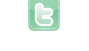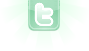## Randomly Generated Map

So, after toying around with Greenfoot for a while, i decided i want to make a game or program that generates a completely random terrain, which the user can then explore. How will i go about doing this? Right now i made a small program thats a bit like it, but generates a fixed amount of each terrain, i want to generate a random amount, but i only know of the method addObject( new object(), 0, 0); and i dont know how to run that method a random number of times... Thanks in advance!
You can get a random number by calling Greenfoot.getRandomNumber(int range); and assign it to a variable... For example...
```//This will make int random_terrain a random number between 0 and 100
int random_terrain = Greenfoot.getRandomNumber(100);

for(random_terrain = random_terrain; random_terrain > 0)
{
addObject(new Object(), int x, int y);
random_terrain--;
}```
Thanks, was having a blonde moment actually... Forgot about the for loop, i for some reason rarely use it... Thanks, its a great help. Now to get cracking on the actual program :)
I suggest a "while" loop instead. The logic is exactly the same but it avoids the unnecessary assignment:
```//This will make int random_terrain a random number between 0 and 100
int random_terrain = Greenfoot.getRandomNumber(100);

while (random_terrain > 0)
{
addObject(new Object(), int x, int y);
random_terrain--;
}```
You could also just write the "for" loop as:
```for( ; random_terrain > 0 ; random_terrain-- )
{
addObject(new Object(), int x, int y);
}```
thanks, sounds good :)
how can i get a random negative integer? Greenfoot.getRandomNumber(-50);?
@w1llis: Greenfoot.getRandomNumber(int n) is from 0 to n-1 numbers. But you can do for any ranges, from "InitialValue" to "FinalValue", like this:
`int randomNumber = Greenfoot.getRandomNumber(FinalValue-InitialValue +1) + InitialValue;`
For examples: From -50 to 70, we have
`int randomNumber = Greenfoot.getRandomNumber(121) -50;`
From 30 to 50:
`int randomNumber = Greenfoot.getRandomNumber(21) +30;`
From -100 to -50:
`int randomNumber = Greenfoot.getRandomNumber(51) -100;`
Good luck!
You could also just get a random number then apply a (-) to it.
```int random = Greenfoot.getRandomNumber(50);
random = -random;```
Thanks Wagnerf and DonaldDuck. I'm slowly re-learning Java :)